• 第二节 函数求导法则


文章目录
1. 函数的和、差、积、商的求导法则2. 反函数的求导法则3. 复合函数的求导法则4. 常数和基本初等函数求导公式总结

1. 函数的和、差、积、商的求导法则
四则运算 如果函数

u

=

u

(

x

)

u=u(x)

及

v

=

v

(

x

)

v=v(x)

都在点

x

x

具有导数 那么它们的和、差、积、商（除分母为零的点外）都在点

x

x

具有导数，且

(

1

)

[

u

(

x

)

±

v

(

x

)

]

′

=

u

′

(

x

)

±

v

′

(

x

)

;

(1)[u(x)\pm v(x)]'=u'(x)\pm v'(x);

(

2

)

[

u

(

x

)

⋅

v

(

x

)

]

′

=

u

′

(

x

)

v

(

x

)

+

u

(

x

)

v

′

(

x

)

;

(2)[u(x)·v(x)]'=u'(x)v(x)+u(x)v'(x);

(

3

)

[

u

(

x

)

v

(

x

)

]

′

=

u

′

(

x

)

v

(

x

)

−

u

(

x

)

v

′

(

x

)

v

2

(

x

)

(

v

(

x

)

≠

0

)

(3)[\dfrac{u(x)}{v(x)}]'=\dfrac{u'(x)v(x)-u(x)v'(x)}{v^2(x)}(v(x)\ne 0)

2. 反函数的求导法则
反函数 如果函数

x

=

f

(

y

)

x=f(y)

在区间

I

y

I_y

内单调、可导且

f

′

(

y

)

≠

0

f'(y)\ne 0

那么反函数

y

=

f

−

1

(

x

)

y=f^{-1}(x)

在区间

I

x

=

{

x

∣

x

=

f

(

y

)

,

y

∈

I

y

}

I_x=\{x|x=f(y),y\in I_y\}

内也可导，且

[

f

−

1

(

x

)

]

′

=

1

f

′

(

y

)

或

d

y

d

x

=

1

d

x

d

y

[f^{-1}(x)]'=\frac{1}{f'(y)}或\frac{dy}{dx}=\cfrac{1}{\cfrac{dx}{dy}}

反函数的导数等于直接函数导数的倒数
3. 复合函数的求导法则
复合函数 如果

u

=

g

(

x

)

u=g(x)

在点

x

x

可导，而

y

=

f

(

u

)

y=f(u)

在点

u

=

g

(

x

)

u=g(x)

可导 那么复合函数

y

=

f

[

g

(

x

)

]

y=f[g(x)]

在点

x

x

可导，且其导数为

d

y

d

x

=

f

′

(

u

)

g

′

(

x

)

或

d

y

d

x

=

d

y

d

u

⋅

d

u

d

x

\frac{dy}{dx}=f'(u)g'(x)或\frac{dy}{dx}=\frac{dy}{du}·\frac{du}{dx}

4. 常数和基本初等函数求导公式总结
常数

(

C

)

′

=

0

(C)'=0

幂函数

(

x

μ

)

′

=

μ

x

μ

−

1

(x^\mu)'=\mu x^{\mu-1}

三角函数

(

sin

⁡

x

)

′

=

cos

⁡

x

(\sin x)'=\cos x

(

cos

⁡

x

)

′

=

−

sin

⁡

x

(\cos x)'=-\sin x

(

tan

⁡

x

)

′

=

sec

⁡

2

x

(\tan x)'=\sec^2x

(

cot

⁡

x

)

′

=

−

csc

⁡

2

x

(\cot x)'=-\csc^2 x

(

sec

⁡

x

)

′

=

sec

⁡

x

tan

⁡

x

(\sec x)'=\sec x\tan x

(

csc

⁡

x

)

′

=

−

csc

⁡

x

cot

⁡

x

(\csc x)'=-\csc x\cot x

指数函数

(

a

x

)

′

=

a

x

ln

⁡

a

(

a

>

0

,

a

≠

1

)

(a^x)'=a^x\ln a(a>0,a\ne1)

(

e

x

)

′

=

e

x

(e^x)'=e^x

对数函数

(

log

⁡

a

x

)

′

=

1

x

ln

⁡

a

(

a

>

0

,

a

≠

1

)

(\log_ax)'=\cfrac{1}{x\ln a}(a>0,a\ne1)

(

ln

⁡

x

)

′

=

1

x

(\ln x)'=\cfrac{1}{x}

反三角函数

(

arcsin

⁡

x

)

′

=

1

1

−

x

2

(\arcsin x)'=\cfrac{1}{\sqrt{1-x^2}}

(

arccos

⁡

x

)

′

=

−

1

1

−

x

2

(\arccos x)'=-\cfrac{1}{\sqrt{1-x^2}}

(

arctan

⁡

x

)

′

=

1

1

+

x

2

(\arctan x)'=\cfrac{1}{1+x^2}

(

a

r

c

c

o

t

)

′

=

−

1

1

+

x

2

\newcommand{\arccot}{\mathrm{arccot}\,}(\arccot)'=-\cfrac{1}{1+x^2}


展开全文高等数学
• 求导公式 导数运输法则 复合函数求导法则 幂指函数求导 取对数后按照符合函数求导法则
求导公式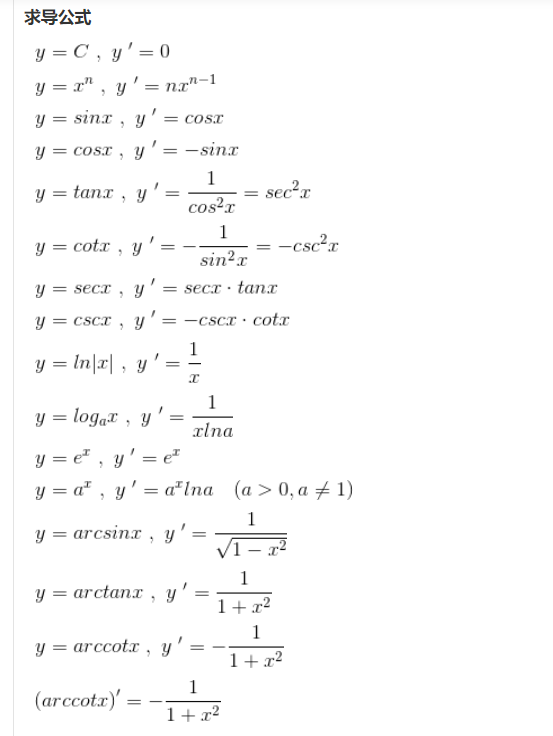导数运输法则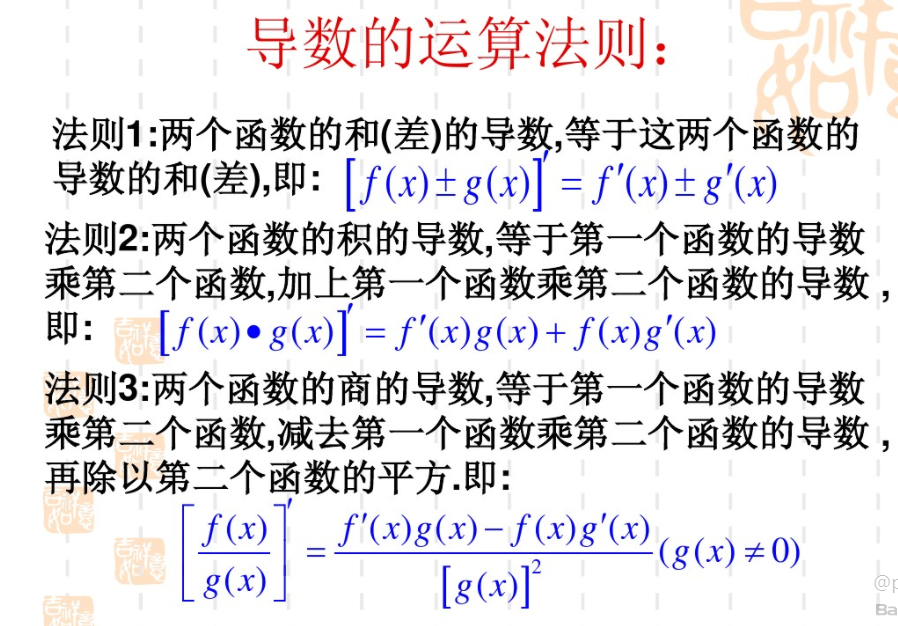复合函数求导法则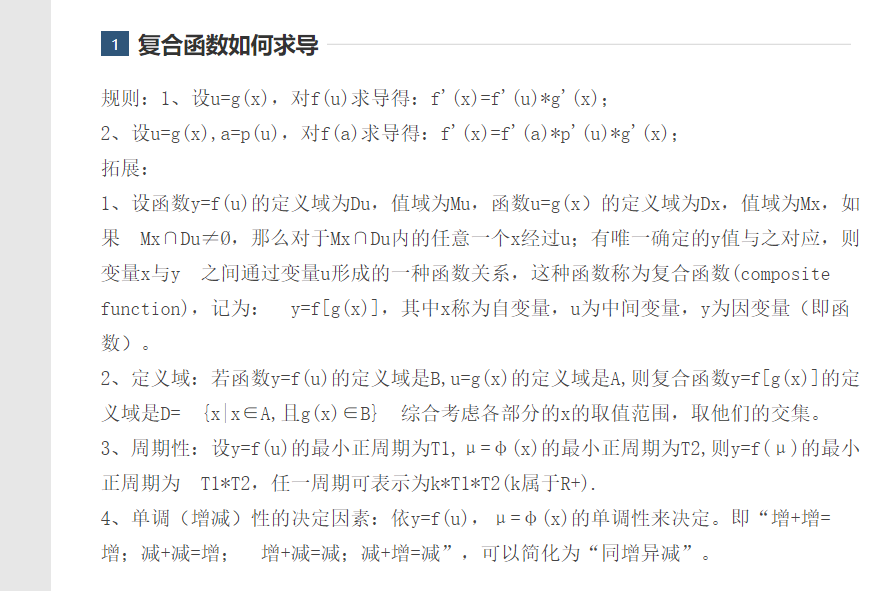幂指函数求导
取对数后按照符合函数求导法则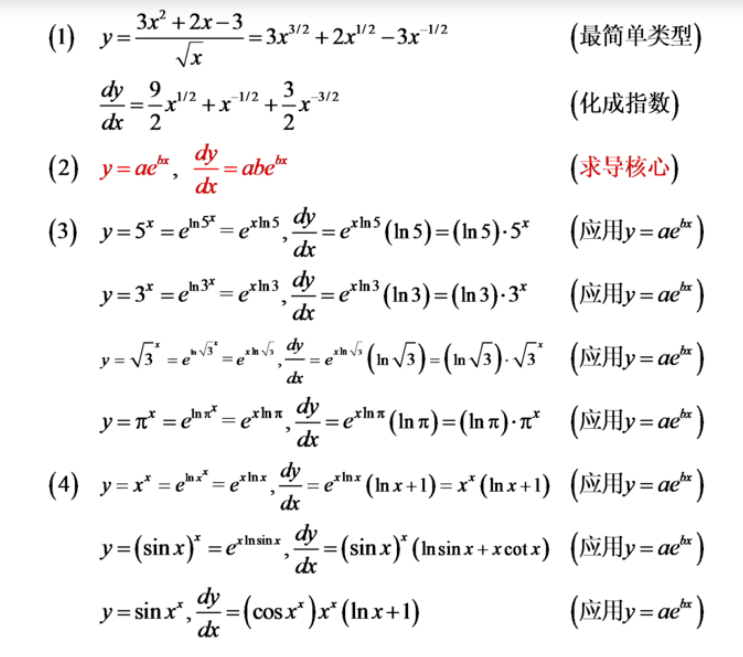二阶导求导方法
二阶导等于对一阶导再次求导
复合隐函数求导
直接法
直接对方程两边求导
公式法
用到偏导数
偏导数
展开全文数学
• 函数在高等数学中经常用于求导，一个函数f(x)求导...（8）反函数求导法则 定理2要证明，你看一看标红的地方，已经证明了， 小学的玩意。下面是课本证明，看看就行了。重点是划红线的地方。 （9）y...
反函数在高等数学中经常用于求导，一个函数f(x)求导不是很好求的情况下或者它的反函数更好求导，非常实用。包括在对数求导的过程中非常好用。
要看对数求导可以看自然数学-导数运算法则和洛必达法则其中就有。
涉及内容多，抓重点就行。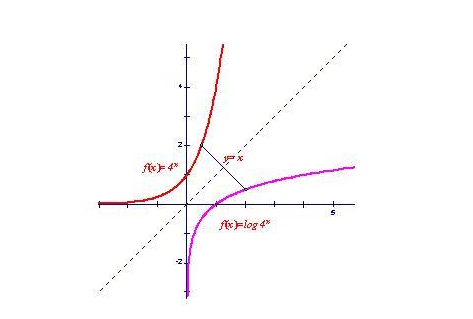反函数与直接函数的关系，别太看文字，看图就行。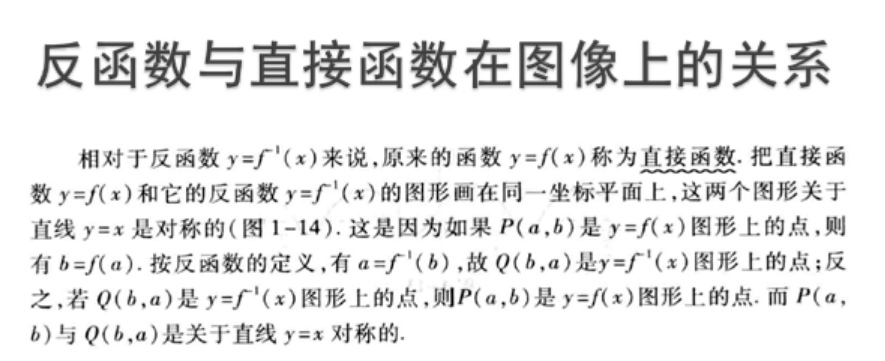展开全文算法
• 数学 - 基本初等函数导数公式及求导法则 三角函数相关运算 指数和对数函数相关运算 对数函数的强大之处在于可以变积为和，变商为差，化幂为系数。在求幂指函数或某些复杂表达式的函数的导数时，将原来的函数...
数学 - 基本初等函数导数公式及求导法则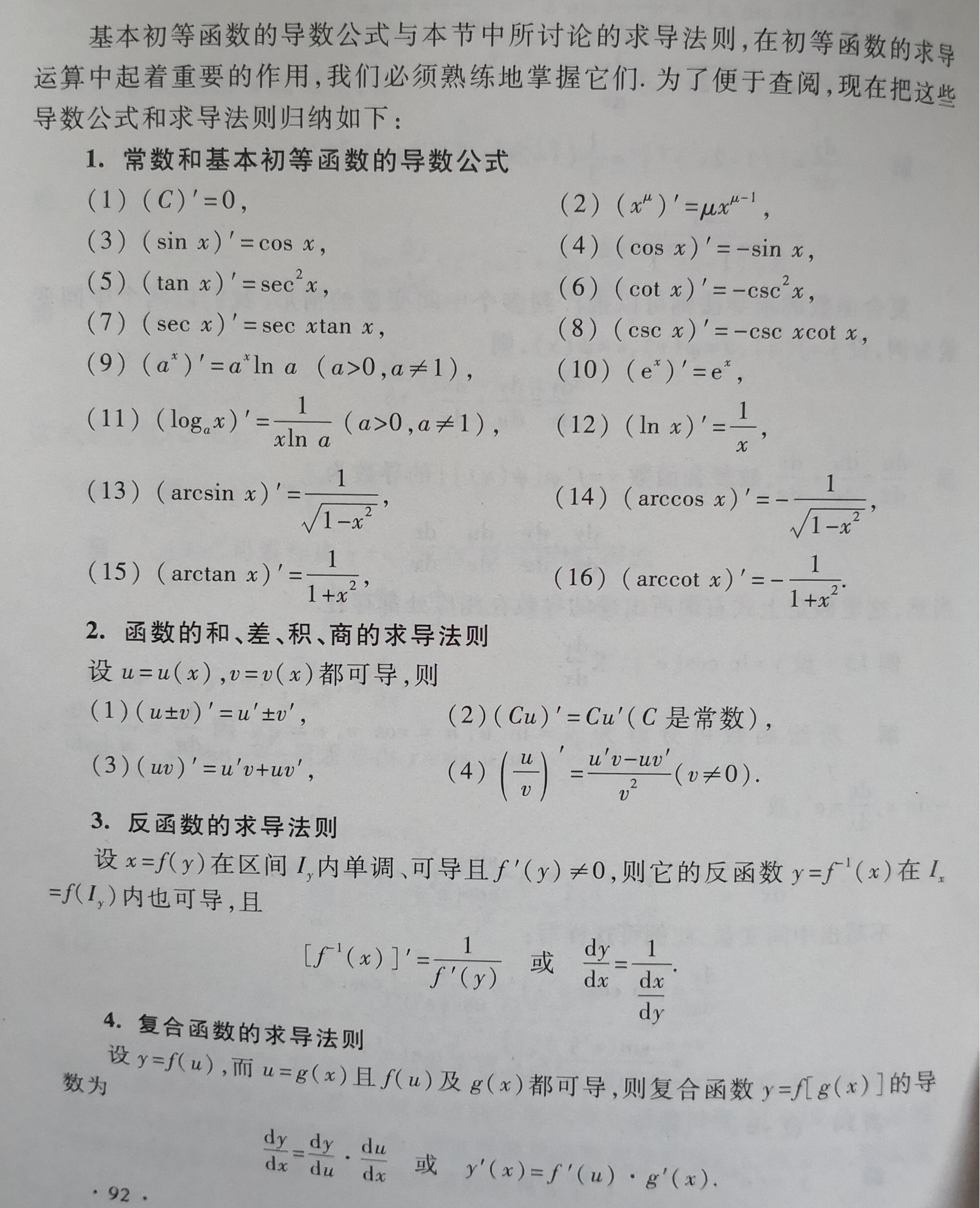三角函数相关运算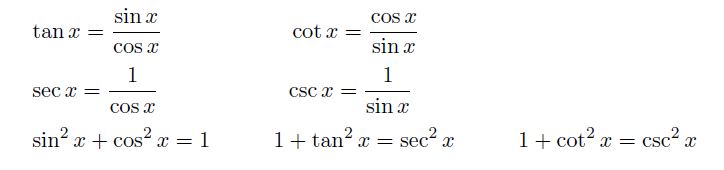指数和对数函数相关运算
对数函数的强大之处在于可以变积为和，变商为差，化幂为系数。在求幂指函数或某些复杂表达式的函数的导数时，将原来的函数转化为对数函数后可方便求导。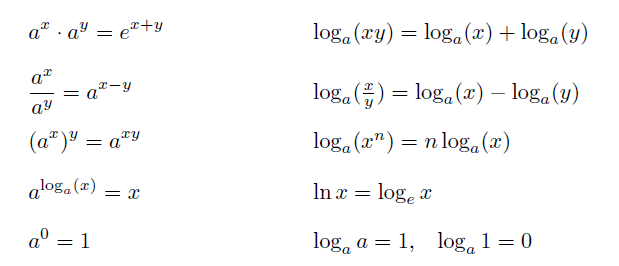隐函数求导
“如果方程F(x,y)=0能确定y是x的函数，那么称这种方式表示的函数是隐函数。一般情况下无法写成y=f(x)这种格式，任何的显函数都可以转化为隐函数，但隐函数不一定能转化为显函数。隐函数求导规则是分别将等式两边对x求导，再解出dy/dx。
例: x2 + y2 = 1，求dy/dx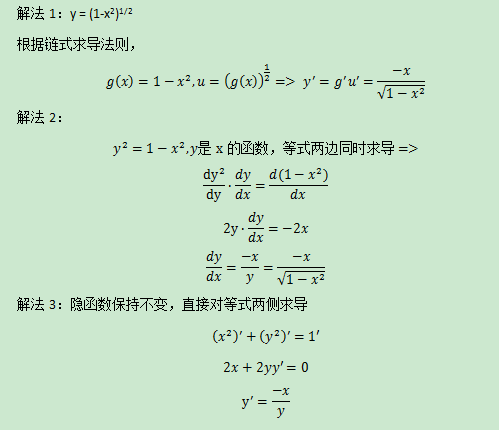参数方程求导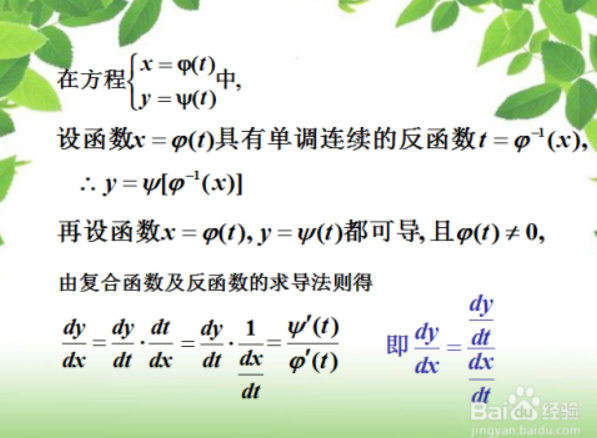幂指函数求导
幂指函数不能直接用初等函数公式求导，一般做法是两边同时取对数，再对x求导。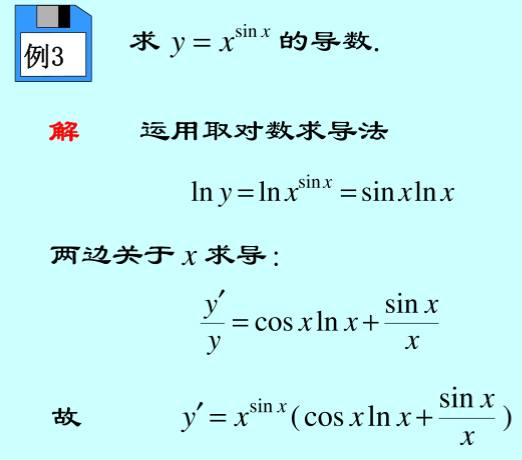某些复杂表达式函数求导
对于某些复杂表达式函数的求导，同样先取对数后再求导。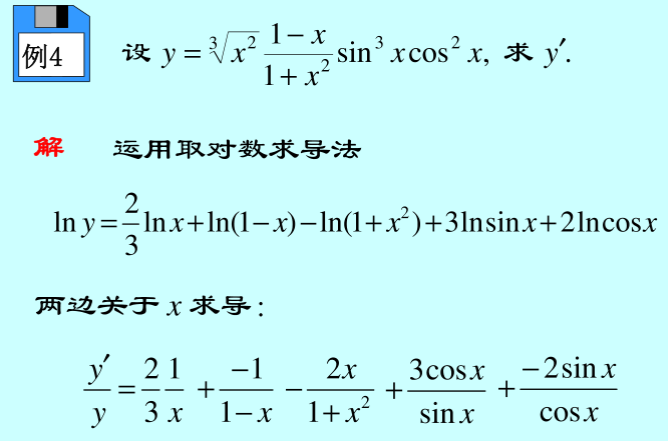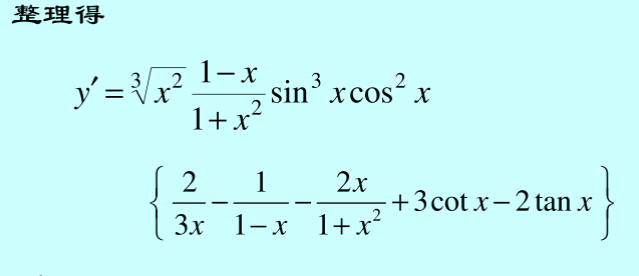Maple求导
初等函数求导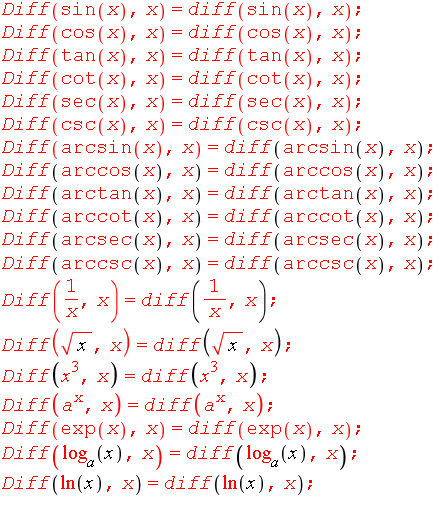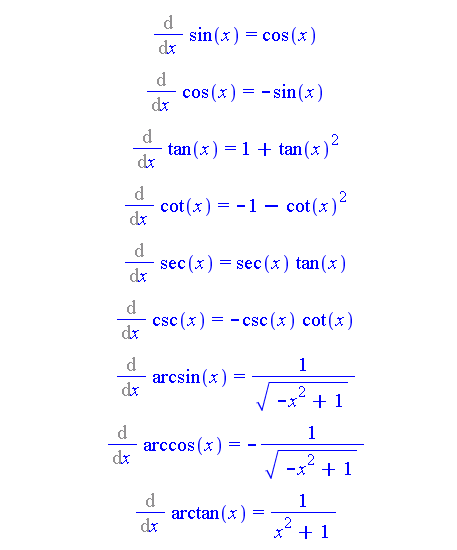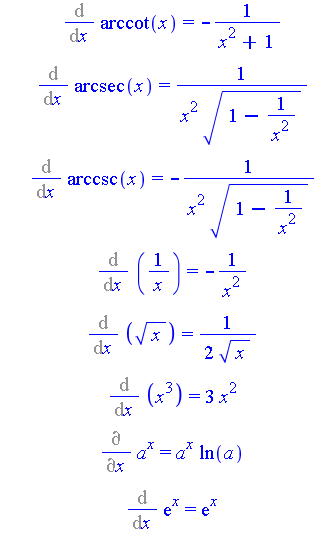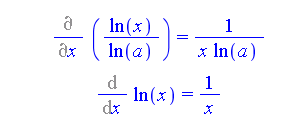隐函数求导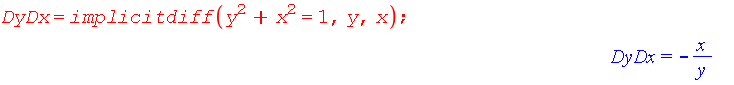参数方程函数求导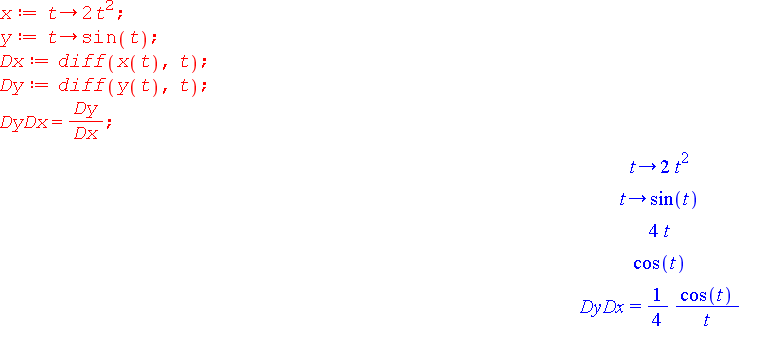展开全文• 指数、对数公式https://wenku.baidu.com/view/69653d53f01dc281e53af0ba.html 求导公式... 复合函数求导法则https://baike.baidu.com/item/复合函数求导法则/15792114 复...数学基础 机器学习
• 求导法则： 三角函数运算 指数和对数函数相关运算 隐函数求导 参数方程求导 幂指函数求导 复杂表达式函数求导算法
• 1.1、分部积分法 关键是选择正确的u,v, 是的后部分积分好算 1.1、选幂函数作为u, du会将被积函数降幂，而v不会改变次数...1.2、选取对数函数或三角函数作为u 1.3、特殊 1.4、兼顾换元法和分部积分法
• 函数$f_{ij}(x)(i,j=1,2,\cdots,n)$在区间$I$内可导，则行列式函数 \begin{equation} f(x)=\begin{vmatrix} f_{11}(x)&f_{12}(x)&\cdots&f_{1n}(x)\\f_{21}(x)&f_{22}(x)&\cdots&f_{2n}(x...
• 2 对数函数与sigmoid 2sigmoid函数求导 3神经网络损失函数求导 1、sigmoid函数​ sigmoid函数，也就是s型曲线函数，如下： 函数：f(z)=11+e−z 函数：f(z)=\frac{1}{1+e^{-z}} 导数：f′(z)=f(z)(1−f(z)) 导数：f'...深度学习
• 2sigmoid函数求导3神经网络损失函数求导 1、sigmoid函数 ​ sigmoid函数，也就是s型曲线函数，如下：  函数：f(z)=11+e−z 导数：f′(z)=f(z)(1−f(z)) ​ 上面是我们常见的...
• 微分1、导数1.1 例题—导数定义求导(important)1.2 单侧导数1.3 例题—判断是否可导2、函数的求导法则2.1 定理一 线性组合求导的传递性2.2 定理二 反函数的求导法则2.2.1 例题—利用反函数求导法则求导2.3 定理三 ...
• ## 链式求导法则

万次阅读 2018-05-30 13:46:16
链式法则是微积分中的求导法则，用以求一个复合函数的导数。所谓的复合函数，是指以一个函数作为另一个函数的自变量。如f(x)=3x，g(x)=x+3，g(f(x))就是一个复合函数，并且g(f(x))=3x+3链式法则(chain rule)：若h(x)...
• §2.1 导数的概念 一、从两个例子来谈导数的概念 ...设动点于时刻在直线上所处的位置为，于是，称此函数为位置函数。该如何定义动点在某一时刻的瞬时速度呢? 考虑从到这一时间间隔，质点从位置移动到，其质高等数学 导数与微分
• 相关函数求导公式 先复习回顾下一些数学基础，帮助推导过程可以更好的理解。下面列举的公式都是，接下来的推导中会用到的，没有涉及到的公式，此处不再列举。 常数项求导 以 e 为底的指数求导公式 ...
• 函数：1.1 从指数函数到sigmoid​ 首先我们来画出指数函数的基本图形：​ 从上图，我们得到了这样的几个信息，指数函数过(0,1)点，单调递增/递减，定义域为(−∞,+∞)，值域为(0,+∞)，再来我们看一下sigmoid函数的...tanh
• 本章主要回顾指数函数和对数函数，指数函数和对数函数求导 回顾指数函数和对数函数的基础知识 eee 的定义和性质 如何对指数函数和对数函数求导 指数函数和对数函数的极限求解 对数函数的微分 指数增长和指数...
• 指数函数求导的代数理解；有关自然常数e的理解！！机器学习 抽象代数 算法
• 令 , 所以 ，俩边求导, 根据复合函数求导法则为： 或者记住 的导数，用复合函数求导推 的导数。但是个人觉得这种做法太讨巧了，而且我也不是总能记住其中一个的导数是什么，一般是一忘就都忘了。理解一个...指数函数 定义
• 机器学习和深度学习中比较重要的内容便是计算图，主流的框架如tensorflow，pytorch都是以计算图为主要框架。...我们先定义表达式（由初等函数构造而成），在表达式构造完成之前不进行计算。完成后，传入自变...
• 函数求导——对等式方程两边同时求导具有怎样的实际意义呢？
• 链式求导法则-微积分高数解答版 看了深度学习的反向计算的链式法则是不是一脸懵，不怕，本文从大学老师的讲解方法让你从根本上理解链式法则 注：本文适合学过高数，但又把知识还给老师的小伙伴。能让你立马回忆...深度学习 算法
• 我们在高考数学中学过指数函数求导公式 f(x)=axf′(x)=axln⁡a(a&amp;amp;amp;amp;amp;amp;amp;gt;0,a≠1)f(x) = a^x\\ f&amp;amp;amp;amp;amp;amp;amp;#x27;(x) = a^x\ln{a}(a&amp;amp;amp;amp;amp...微积分
• 宜城教育资源网www.ychedu.comx的a次方的导数图像-导数的求导法则-x的a次方求导公式用定义推导导数的求导法则1.求导的线性：对函数的线性组合求导，等于先对其中每个部分求导后再取线性组合。2.两个函数的乘积的导...
• 指数函数与对数函数4.1 指数函数回顾4.2 对数函数回顾4.3 指数函数与对数函数互为反函数4.4 对数法则 4.指数函数与对数函数 4.1 指数函数回顾 底数指数底数^{指数}底数指数 4.2 对数函数回顾 y=2x=8y=2^x=8y=2x=8...指数函数 双曲函数 数学
• 第五章Neural Networks由网神主讲，精彩内容有：神经网络做回归和分类的训练目标函数、BP误差后向传播的链式求导法则、正则化、卷积网络等。神经网络 正则化 卷积网络
• 法则5（反函数求导法则）如果函数在区间内单调连续,且在该区间内处处有不等于0的导数，那么它的反函数在相应区间内也处处可导,即存在，并且   也可写为  或  这个等式还可以简单地说成反函数的导数等于原...